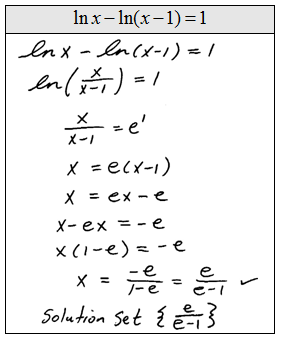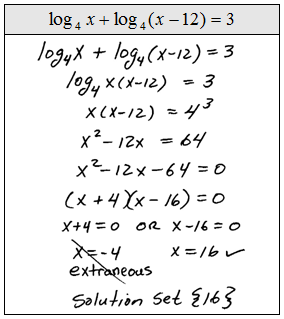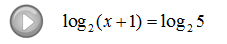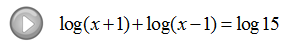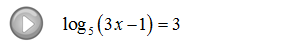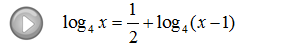## Algebra

### Solving Logarithmic Equations

Use the one-to-one property for logarithms to solve logarithmic equations.

If we are given an equation with a logarithm of the same base on both sides we may simply equate the arguments.

Step 1: Use the rules of exponents to isolate a logarithmic expression (with the same base) on both sides of the equation.
Step 2: Set the arguments equal to each other.
Step 3: Solve the resulting equation.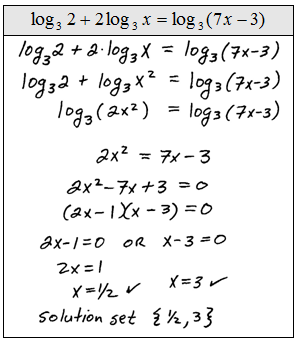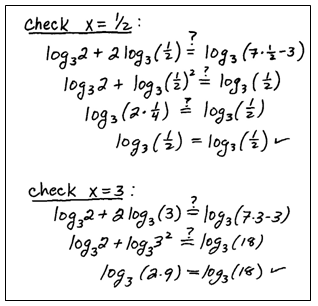Be sure to check to see if the solutions that you obtain solve the original logarithmic equation. In this study guide we will put a check mark next to the solution after we determine that it really does solve the equation. This process sometimes results in extraneous solutions so we must check our answers.
Solve.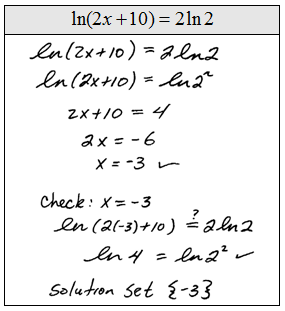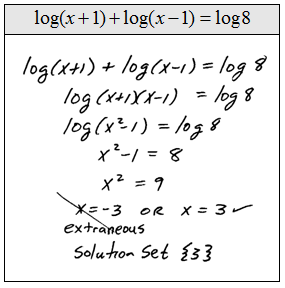Of course, equations like these are very special.  Most of the problems that we will encounter will not have a logarithm on both sides. The steps for solving them follow.

Step 1: Use the properties of the logarithm to isolate the log on one side.
Step 2: Apply the definition of the logarithm and rewrite it as an exponential equation.
Step 3: Solve the resulting equation.If the answer to the logarithmic equation makes the argument negative then it is extraneous.  This does not preclude negative answers. We must be sure to check all of our solutions.

Instructional Video: Solving Logarithmic Equations

Solve.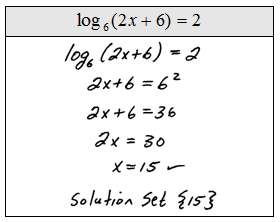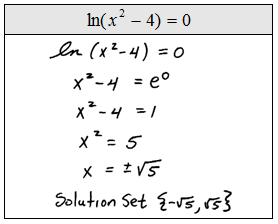Tip:  Not all negative solutions are extraneous!  Look at the previous set of problems and see that some have negative answers. The check mark indicates that we actually plugged the answers in to see that they do indeed solve the original.  Please do not skip this step, extraneous solutions occur often.